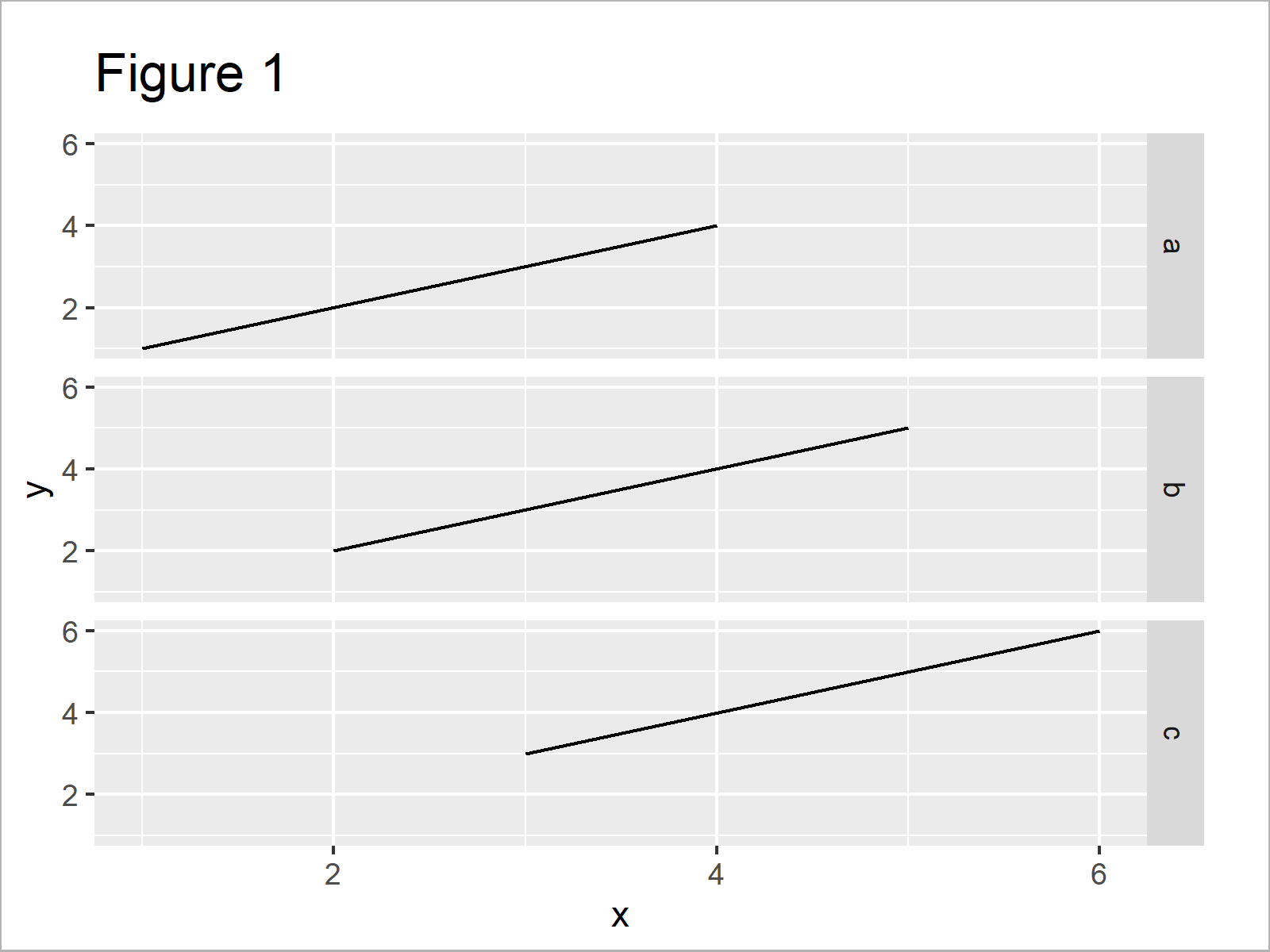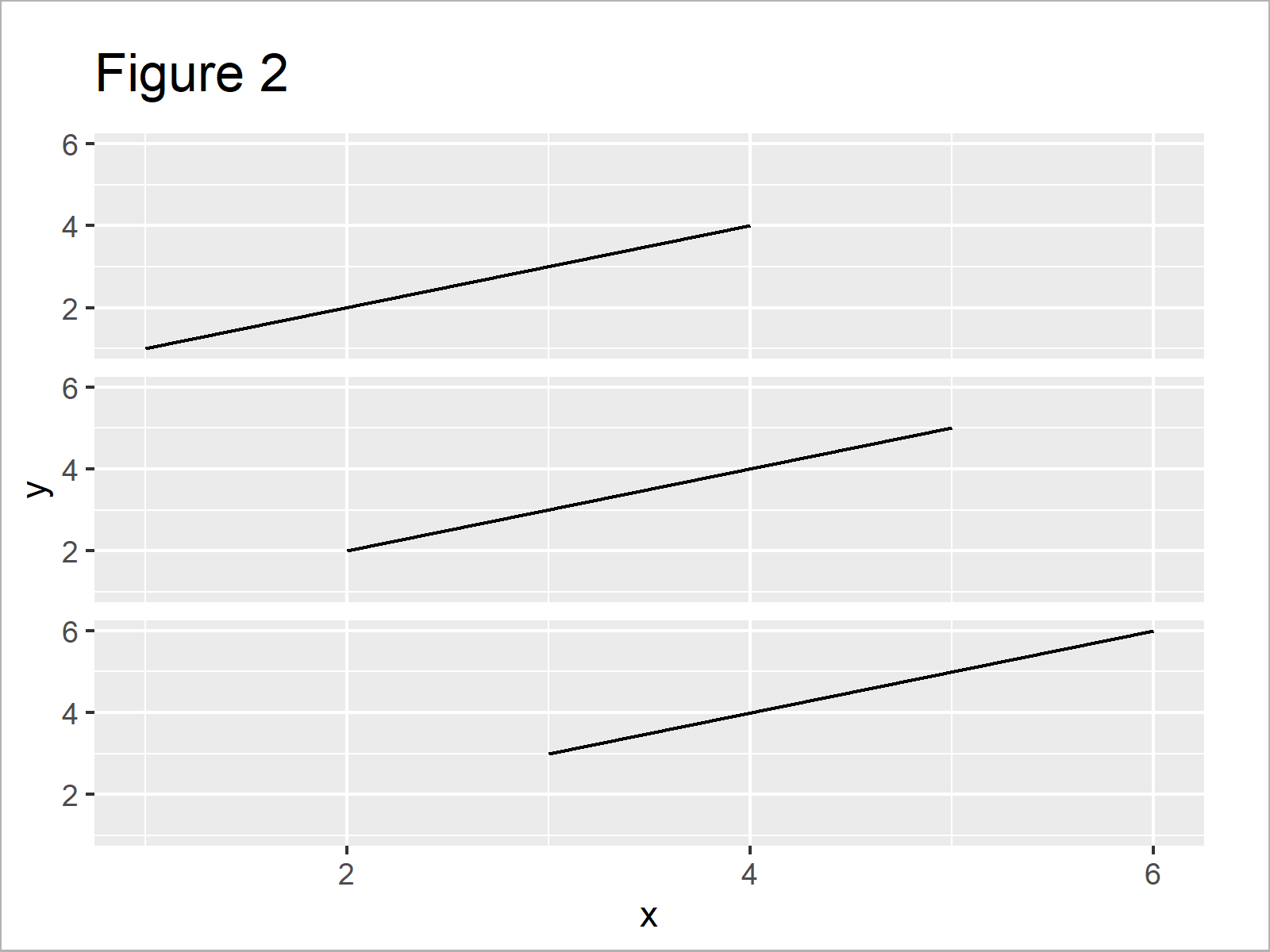# Remove Labels from ggplot2 Facet Plot in R (Example)

This tutorial explains how to delete all labels and boxes from a ggplot2 facet plot in R programming.

The article will contain one example for the creation of facet plots without labels. To be more precise, the page consists of the following content blocks:

Let’s start right away…

## Example Data, Add-On Packages & Basic Plot

First, let’s create some example data in R:

```data <- data.frame(x = 1:6,         # Create example data
y = 1:6,
group = letters[1:3])
data                                # Display example data```As you can see based on Table 1, the example data is a data frame consisting of six lines and three columns.

We also need to install and load the ggplot2 package, if we want to use the functions that are included in the package:

```install.packages("ggplot2")         # Install ggplot2 package

As next step, we can plot our data in a ggplot2 facet graphic:

```ggp <- ggplot(data, aes(x, y)) +    # Create ggplot2 facet plot
geom_line() +
facet_grid(group ~ .)
ggp                                 # Draw ggplot2 facet plot```In Figure 1 it is shown that we have created a line plot with three different panel windows. On the right side of each facet, a label is shown (i.e. a, b and c).

## Example: Remove Labels from ggplot2 Facet Plot Using strip.text.y & element_blank

In this example, I’ll explain how to drop the label box and the labels from our ggplot2 facet plot.

For this, we can use the theme function, in and within the theme function we have to specify the strip.text.y argument to be equal to element_blank().

Check out the following R syntax:

```ggp +                               # Remove labels from facet plot
theme(strip.text.y = element_blank())```After executing the previous code the ggpot2 facet graph without labels shown in Figure 2 has been created.

## Video & Further Resources

I have recently published a video on my YouTube channel, which shows the content of this tutorial. You can find the video below:

Please accept YouTube cookies to play this video. By accepting you will be accessing content from YouTube, a service provided by an external third party.If you accept this notice, your choice will be saved and the page will refresh.

In addition, you may want to read some of the related articles of my homepage. I have published numerous articles already:

You have learned in this article how to get rid of facet plot label boxes in the R programming language. Note that the same R syntax could be applied in case of the facet_wrap instead of the facet_plot function. If you have any further questions and/or comments, please let me know in the comments section below.

Subscribe to the Statistics Globe Newsletter